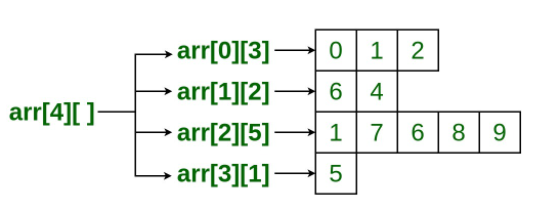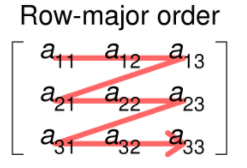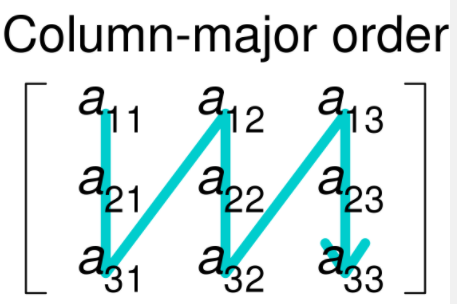By now you know how regular one-dimensional arrays work. What if each element of a one-dimensional array was an array? You can use two-dimensional arrays which are actually arrays of arrays whenever it is useful for data to be organized in rows and columns like a seating chart or table. It might be easy to imagine two-dimensional arrays as a stack of one-dimensional arrays. Each row is a 1D array.

#### Declaration and Instantiation

Declaring and creating a two-dimensional array is simply an extension of what you know about how to perform these actions with one-dimensional arrays. Notice the extra sets of brackets!
The general form becomes:

`type[][] name = new type[numRows][numCols];`

```//1D
int[] my1d = new int;
//2D of 4 rows 2 columns
//this means that each row has a length of 2
int[][] my2d = new int;
//1D
int[] my1d2 = { 1, 2, 3};
//2D
int[][] my2d2 = { {1, 2, 3}, {4, 5, 6} };```

Practice Creating Arrays in the Practice Section!

#### `length`

To get the number of rows of a 2D array you call the length property on the 2D array.

```int[][] my2d2 = { {1, 2, 3}, {4, 5, 6} };
System.out.println("rows:"+my2d2.length);
```
To get the number of columns you have to access a specific row (which is a 1D array) and then access the length property.
```int[][] my2d2 = { {1, 2, 3}, {4, 5, 6} };
System.out.println("cols:"+my2d2.length);
```
You can have jagged 2D arrays where each row might be different lengths, but this is not tested on the AP Exam.
```int[][] my2d2 = { {1, 2, 3}, {4, 6} };
System.out.println("col0:"+my2d2.length);
System.out.println("col1:"+my2d2.length); ///The code above will print 3 and then 2 because the first row of the 2D array has 3 elements and the second row has 2 elements.
```
Since 2D arrays are a stack of 1D arrays so it makes sense that if you go to a particular row, you are simply working with a 1D array!

### Jagged ArraysJagged arrays like the one shown are not tested on the AP exam. The individual rows can actually be of different lengths in the given picture. I suppose if you wanted to save memory and each row did not need to be the same length, a jagged array may help. Can you think of other reasons to use jagged arrays?

#### Traversals

A typical traversal for a 2D array will visit all the rows in an outer for loop and visit each column of the row in the inner for loop. You can read it as "For each row, visit each column of the row".

### Row-Major TraversalA row-major traversal in programming is when you visit all the elements of a row first before moving to the next row.
In code that would look like this:
for(int row = 0; row < my2D.length; row++){
for(int col = 0; col < my2D[row].length; col++){
//access elements at row,col
}
}

`Column-Major Traversal`A column-major traversal in programming is when you visit all the elements of a column first before moving to the next column.
In code that would look like this:
for(int col= 0; col< my2D.length; col++){
for(int row= 0; row< my2D.length; row++){
//access elements at row,col
}
}

Traversal to sum all the elements of a 2D array of integers.

```int sum = 0;
for(int r = 0; r < arr2D.length; r++){
for(int c = 0; c < arr2D[r].length; c++){
sum += arr2D[r][c];
}
}```

Traversal to sum all the diagonal elements of a square (same # of columns and rows) 2D array of integers from the top-left corner to the bottom-right corner.

```int sum = 0;
for(int i = 0; i < arr2D.length; i++){
sum += arr2D[i][i];
}
}
Since you're accessing diagonal elements, row and col is the same so only one variable is required. For example, arr2D is the first element and arr2D is the second element of the diagonal that starts from the top-left to the bottom-right of the 2D array. Notice how the indexes are the same for two sets of square brackets!```

#### Enhanced For Loop

You will typically find enhanced for-loops in problem definitions especially in AP-Exam style questions. These types of loops make the code easier to read. You should work through the practice problems and practice traversing 2D arrays.

```for(int[] row : my2DArray){
//this can be read as "for each 1d array row in the 2D array called my2DArray   //this enhanced for-loop or a foreach loop will visit each row of the 2D array for you   //Then what? Well, you have already learned how to work with 1D arrays so now you can write   //any code that is valid for a 1D array such as a traversal or data manipulation
for(int el: row){
//here is another enhanced for-loop that now traverses the row 1d array!
//this can be read as "for each element el in the 1D array called row"
}
}```